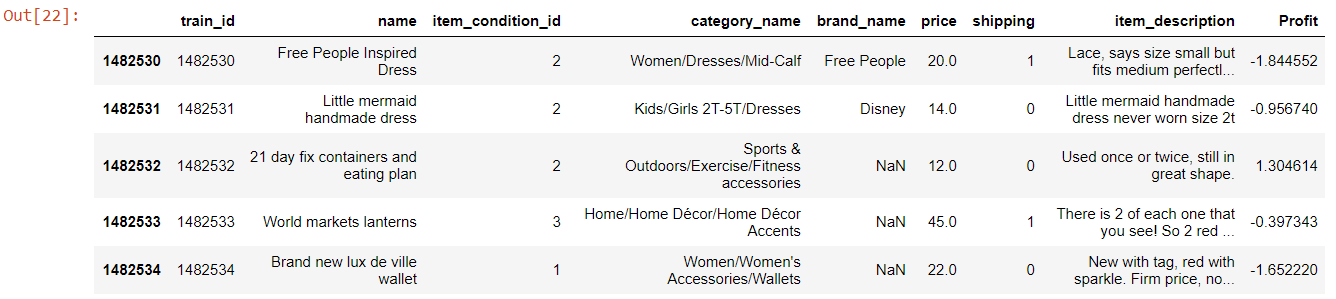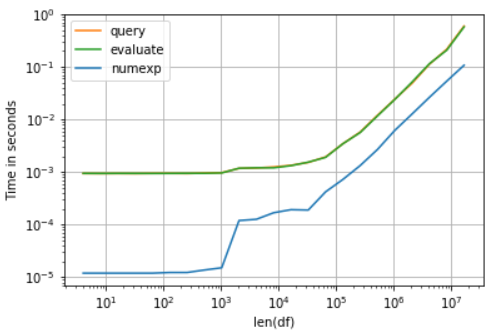# How to Optimize your Pandas Code

Working with large dataset has always been a challenging and daunting task. The data is growing everyday but the resources and computational power required to process this big data is limited. In last few year we have seen Pandas growing popularity within the data science fraternity and it is one of the widely used python library for data cleaning and processing. However it still works on a single core and every data lover wants to process their data as fast as he or she can and this cannot be achieved unless you know how to write clean, fast and better code in Pandas.

Few months back I ask one of my Team member a fresh college passout to clean-up a dataset and pre-process to develop a EDA report for the business problem in hand that we are trying to solve with this data. There were 8 million records and more than 100 columns in this data and he was using all the technique he learnt from all the MOOCS which he studied as a beginner to data science. After a 2-3 days he came running to me and asked why do we need to use pandas if it is being that slow. Why can’t we just use excel and process this data in chunks and then dump to some SQL table. After that I explained him all day why pandas and how can we optimize our pandas code and what are the common mistakes he was making while data cleaning and what’s the right way to implement his learning efficiently. So here thru this blog I want to summarize my explanation and share with everyone.

Here is the snap shot of the data which I have used to explain:

``````df.head()
``````Our objective here is to Remove Special Character ‘&’ from the category_name column of this data set.

We are going to use the following three methods and record the performance of each of these methods.

a) Basic Looping

b) Apply

c) Numba

This is a basic function defined to strip the special character “&” from the category_name column.

``````def remove_special_char(x):
return x.replace('\&','')

@numba.jit
def remove_special_char_with_numba(x):
return x.replace('\&','')
``````

Basic Looping

We all have at one point of time use this basic looping to iterate through each row of the dataframe and pass through a function to get the desired result. This is an easy way to get the things done but it’s the most slowest approach when working with large dataset. This should be the last resort when you are left with no other option than basic looping.

Pandas follows a “Convention Over Configuration” approach in its API design. This means that the same API has been fitted to cater to a broad range of data and use cases.

iteration shines when working with small rows of DataFrames, mixed datatypes, and regular expressions. The speedup you get depends on your data and your problem, so your mileage may vary. The best thing to do is to carefully run tests and see if the payout is worth the effort.

I am using the %timeit magic function to capture the time it takes to execute a python statement or expression

it took around 2.19 sec with a Standard Deviation of 119 ms. The basic looping doesn’t uses any of the pandas or numpy optimization techniques and hence one of the inefficient way to process large data sets.

``````# Looping in Pandas %timeit df\['category\_name'\] = remove\_special\_char(df)
``````

2.19 s ± 119 ms per loop (mean ± std. dev. of 7 runs, 1 loop each)

Apply

This is much better than the basic looping because the object passed to the function is Pandas series object with index as rows (axis=0) or Dataframe column (axis=1) and it returns a new Series or DataFrame object. Even observed the memory consumption was high when using apply over 1.4 million rows. However under the hood this also iterates through the rows of the dataframe but with internal implementation optimization. The result was far better with apply method than the basic looping and an improvement of almost almost 80% is seen, which is pretty fast.

``````# Using Apply
%timeit df['category_name']=df['category_name'].apply(remove_special_char_without_numba)
``````

437 ms ± 34.1 ms per loop (mean ± std. dev. of 7 runs, 1 loop each)

Numba

A recent alternative to statically compiling Cython code, is to use a dynamic jit-compiler, Numba.

As per it documentation, Numba works by generating optimized machine code using the LLVM compiler infrastructure at import time, runtime, or statically (using the included pycc tool). Numba supports compilation of Python to run on either CPU or GPU hardware, and is designed to integrate with the Python scientific software stack.

In order to compile the code with Numba just take the regular python code and annotate with the numba jit - just in time @jit decorator.

We have seen a drastic improvement using numba and the overall time to execute the same code is reduced to 87.2 ms

``````# Using numba
%timeit df['category_name'] = remove_special_char_with_numba(df['category_name'])
``````

87.2 ms ± 1.83 ms per loop (mean ± std. dev. of 7 runs, 1 loop each)

Conclusion

You can use basic looping and apply when performance is not of much concern and you have smaller dataset to process.

Numba will be a benefit for functions which has a Run time is primarily due to NumPy array element memory access or numerical operations (integer or float) more complex than a single NumPy function call and Functions which work with data types that are frequently converted by NumPy functions to int64 or float64 for calculations (like int8 and int16).

# Highly Efficient Pandas Function

Pandas eval and query

Pandas eval is used for expression evaluation of Series and DataFrame objects and it is faster because there are no overheads wrt indexing alignment, NaNs, and mixed dtypes. it evaluates the boolean and arithmetic operations which must be passed as String with the speed of C without costly allocation of intermediate arrays. it relies on the Numexpr package, which is fast numerical expression evaluator for NumPy.

pd.eval is the most important function. The df.eval and df.query call pd.eval under the hood. Behaviour and usage is more or less consistent across the three functions, with some minor semantic variations.

As per the documentation:

The result of the evaluation of this expression is first passed to DataFrame.loc and if that fails because of a multidimensional key (e.g., a DataFrame) then the result will be passed to DataFrame.getitem().

Following are the Valid eval expression and will be evaluated by the engine:

``````pd.eval("df1 > df2")
pd.eval("df1 > 5")
pd.eval("df1 < df2 and df3 < df4")
pd.eval("df1 in \[1, 2, 3\]")
pd.eval("1 < 2 < 3")
``````

engine=… argument

There are two options for this argument- numexpr (the default) and python. The numexpr option uses the numexpr backend which is optimized for performance.

With ‘python’ backend, your expression is evaluated similar to just passing the expression to python’s eval function. You have the flexibility of doing more inside expressions, such as string operations.

A list detailing all the supported features and syntax can be found in the Documentation and a detailed Stackoverflow Answer

``````import pandas as pd
nrows, ncols = 100000, 2
rng = np.random.RandomState(42)
df1, df2, df3 = (pd.DataFrame(rng.rand(nrows, ncols))
for i in range(3))
df1.columns = ['start','end']
``````
``````%timeit df1 + df2 + df3
``````

102 ms ± 10.3 ms per loop (mean ± std. dev. of 7 runs, 10 loops each)

``````%timeit pd.eval('df1 + df2 + df3')
``````

60.3 ms ± 5.05 ms per loop (mean ± std. dev. of 7 runs, 10 loops each)

eval almost took half of the time to execute here

Query

This uses the top-level pandas.eval() function to evaluate the passed query.

``````df.query() is basically df[df.eval()]
``````

It also uses numexpr library. You can read more about this function and how it works under the hood in the official pandas documentation here

Typically, query (as the name suggests) is used to evaluate conditional expressions (i.e., expressions that result in True/False values) and return the rows corresponding to the True result. The result of the expression is then passed to loc (in most cases) to return the rows that satisfy the expression. According to the documentation,

This key difference between the two, as mentioned above is how they handle the expression result. This becomes obvious when you actually run an expression through these two functions. For example, consider

``````%timeit df1.query('start < 0.5 and end < 0.5')
``````

4.74 ms ± 800 µs per loop (mean ± std. dev. of 7 runs, 100 loops each)

``````%timeit df1.loc[(df1['start'] < 0.5) & (df1['end'] < 0.5)]
``````

4.33 ms ± 353 µs per loop (mean ± std. dev. of 7 runs, 100 loops each)

You can see loc and Query has almost same performance because The Query result of the evaluation of the expression is first passed to `DataFrame.loc` and if that fails because of a multidimensional key (e.g., a DataFrame) then the result will be passed to  `DataFrame.__getitem__()`.

Numexpr

It is a Fast numerical expression evaluator for NumPy. it uses less memory compared to any other methods discussed above and way faster than any of these methods. it performs well on large array because it doesn’t access memory for intermediate results and uses cache utilization and hence reduces the memory access. This is the main reason Numexpr gets the best of your machine computing capability for array operations on very large datasets.

Here is a comparison of eval, Query, Numpy Vectorization, Numexpr

``````import numexpr as nex

np.random.seed(125)
N = 100000
df = pd.DataFrame({'A':np.random.randint(10, size=N)})

def ne(df):
x = df.A.values
return df[nex.evaluate('(x > 5)')]

print("===Numexpr===")
%timeit (ne(df))

print("===Query===")
%timeit df.query('A > 5')

print("===Eval===")
%timeit df[df.eval('A > 5')]
``````
``````> \===Numexpr===
> 1.55 ms ± 130 µs per loop (mean ± std. dev. of 7 runs, 1000 loops each)
``````
``````> \===Query===
> 3.06 ms ± 290 µs per loop (mean ± std. dev. of 7 runs, 100 loops each)
``````
``````> \===Eval===
> 3.57 ms ± 804 µs per loop (mean ± std. dev. of 7 runs, 100 loops each)
``````

Performance graph for the time of execution for these three functions:I am using perfplot to draw these performance graphs. You can see that Query and Eval took exactly same time and that’s the reason they are overlapping each other in the graph. However Numexpr seems to be a clear winner here. The least time taken is 634ms with a standard deviation of 22.4 ms

Final Words

Pandas is a beautiful library and I have used it since it’s first release and really enjoyed working with it so far. Anyone can learn the art of working with Pandas efficiently once they learn the optimization techniques to write concise, fast and readable Pandas code.